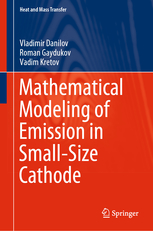• A
• A
• A
• ABC
• ABC
• ABC
• А
• А
• А
• А
• А
Regular version of the site
Menu

## Mathematical Modeling of Emission in Small-Size Cathode

Springer, 2020.
Danilov V., Gaydukov R., Vadim Kretov.

This book deals with mathematical modeling, namely, it describes the mathematical model of heat transfer in a silicon cathode of small (nano) dimensions with the possibility of partial melting taken into account.  This mathematical model is based on the phase field system, i.e., on a contemporary generalization of Stefan-type free boundary problems. The approach used is not purely mathematical but is based on the understanding of the solution structure (construction and study of asymptotic solutions) and computer calculations. The book presents an algorithm for numerical solution of the equations of the mathematical model including its parallel implementation. The results of numerical simulation concludes the book. The book is intended for specialists in the field of heat transfer and field emission processes and can be useful for senior students and postgraduates.​